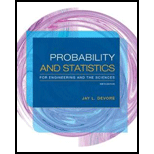# For customers purchasing a refrigerator at a certain appliance store, let A be the event that the refrigerator was manufactured in the U.S., B be the event that the refrigerator had an icemaker, and C be the event that the customer purchased an extended warranty. Relevant probabilities are P ( A ) = .75 P ( B | A ) = .9 P ( B | A ′) = .8 P ( C | A ∩ B ) = .8 P ( C | A ∩ B ′) = .6 P ( C | A ′ ∩ B ) = .7 P ( C | A ′ ∩ B ′) = .3 a. Construct a tree diagram consisting of first-, second-, and third-generation branches, and place an event label and appropriate probability next to each branch. b. Compute P ( A ∩ B ∩ C ). c. Compute P ( B ∩ C ). d. Compute P ( C ). e. Compute P ( A | B ∩ C ), the probability of a U.S. purchase given that an icemaker and extended warranty are also purchased.### Probability and Statistics for Eng...

9th Edition
Jay L. Devore
Publisher: Cengage Learning
ISBN: 9781305251809### Probability and Statistics for Eng...

9th Edition
Jay L. Devore
Publisher: Cengage Learning
ISBN: 9781305251809

#### Solutions

Chapter
Section
Chapter 2.4, Problem 63E
Textbook Problem

## Expert Solution

### Want to see the full answer?

Check out a sample textbook solution.See solution

### Want to see this answer and more?

Experts are waiting 24/7 to provide step-by-step solutions in as fast as 30 minutes!*

See Solution

*Response times vary by subject and question complexity. Median response time is 34 minutes and may be longer for new subjects.